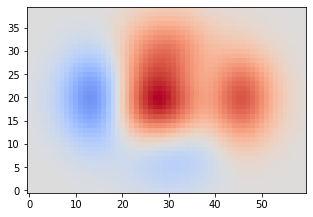# Using Cell Metadata to Select a Thumbnail§

If the nbsphinx-thumbnail cell tag is not enough, you can use cell metadata to specify more options.

The last cell in this notebook has this metadata:

{
"nbsphinx-thumbnail": {
"tooltip": "This tooltip message was defined in cell metadata"
}
}


If there are multiple outputs in the selected cell, the last one is used. See Choosing from Multiple Outputs for how to select a specific output.

:

import matplotlib.pyplot as plt
import numpy as np

:

plt.rcParams['image.cmap'] = 'coolwarm'
plt.rcParams['image.origin'] = 'lower'


Some example data stolen from https://matplotlib.org/examples/pylab_examples/pcolor_demo.html:

:

x, y = np.meshgrid(np.arange(-3, 3, 0.1), np.arange(-2, 2, 0.1))
z = (1 - x / 2 + x ** 5 + y ** 3) * np.exp(-x ** 2 - y ** 2)

:

zmax = np.max(np.abs(z))

:

fig, ax = plt.subplots(figsize=[5, 3.5])
ax.imshow(z, vmin=-zmax, vmax=zmax)

:

<matplotlib.image.AxesImage at 0x7f2dd6ef1910>